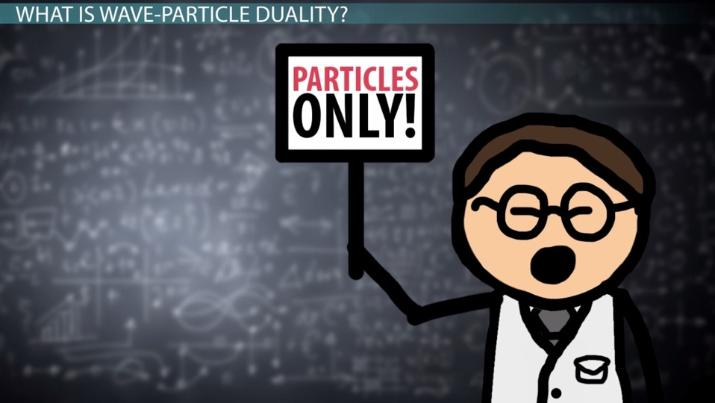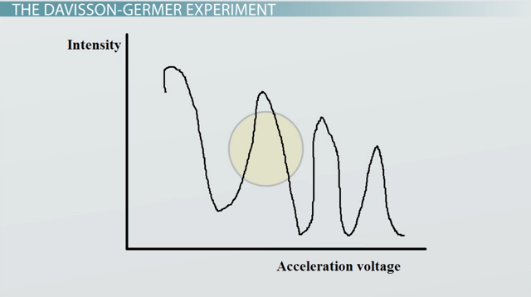# Wave-Particle Duality & the Davisson-Germer Experiment

Lesson Transcript
Instructor: David Wood

David has taught Honors Physics, AP Physics, IB Physics and general science courses. He has a Masters in Education, and a Bachelors in Physics.

After watching this video, you should be able to explain what wave-particle duality is, explain how the Davisson-Germer experiment contributed to our evidence for it, and use the de Broglie equation to solve problems. A short quiz will follow.

## What Is Wave-Particle Duality?

Wave-particle duality is the idea that light and sub-atomic particles can behave both as waves but also as particles. Therefore, the two states are not mutually exclusive. A scientist called de Broglie introduced the idea in 1923, and it formed his doctoral dissertation.

This might be hard to imagine. How could light be both a wave AND a particle? And indeed, physicists found it difficult to accept, too. What we needed to be sure of wasn't just mathematics, but we needed actual experimental evidence. We already knew from the 19th century that light could behave as a wave -- that it exhibited reflection, refraction, and diffraction. These features couldn't be explained any other way. Then, Einstein's photoelectric effect experiment showed that light could also behave like a particle -- it turned out that light contains packets of energy, or 'quanta,' of a particular size. These days we call these packets 'photons.'

But that's just about light. To really pin down the more fundamental idea of wave-particle duality, we needed to also show that not only could light behave as a particle, but particles could also behave as waves.An error occurred trying to load this video.

Try refreshing the page, or contact customer support.

Coming up next: The de Broglie Hypothesis: Definition & Significance

### You're on a roll. Keep up the good work!

Replay
Your next lesson will play in 10 seconds
• 0:00 Wave-Particle Duality
• 1:15 The Davisson-Germer Experiment
• 2:13 The de Broglie Wavelength
• 3:08 Example Calculation
• 4:19 Lesson Summary
Save Save

Want to watch this again later?

Timeline
Autoplay
Autoplay
Speed Speed

## The Davisson-Germer Experiment

In 1928, Clinton Davisson did exactly that in what would later be named the Davisson-Germer experiment. In his experiment, he fired electrons at a solid crystal lattice. Some electrons would reflect off the outermost layer of the crystal, while others would reflect off layers further inside the crystal. The electrons that reflected from the lattice were analyzed and turned out to have light and dark fringes in this pattern:What did the pattern mean? Well, the electrons that bounced off different layers had interfered with each other, just like waves. By different electrons penetrating at different distances, this created a path difference between them. Wherever a peak met a peak or a trough met a trough, he saw a bright spot, called constructive interference. Where a peak met a trough, he saw a dark spot, called destructive interference. Electrons, it seemed, could behave as waves.

## The de Broglie Wavelength

Now that it had been shown that particles and waves were one and the same thing, it was time to put some mathematics to it. De Broglie did this as part of his dissertation. He suggested that if something was a particle and a wave, then it must have both a wavelength (like a wave) and a momentum (like a particle), and that these things must be related to one another.

According to his equation, the de Broglie wavelength, or lambda, of an object or particle, measured in meters, would be equal to Planck's constant, h, which is just a number that is always equal to 6.63 x 10^-34, divided by the momentum of the object or particle, p, measured in kg m/s.

We can also expand on this by remembering the basic equation for momentum, an equation which states that the momentum of an object or particle is equal to the mass, measured in kilograms, multiplied by the velocity, measured in meters per second.

## Example Calculation

We can try this experiment by calculating the wavelength of an everyday object. For example, a Mini Cooper. What is the wavelength of a 1,000 kilogram Mini Cooper moving at 30 m/s?

First of all, we should write out what we know. We have the mass, m, which is 1,000, and the velocity, v, which is 30. We also know that Planck's constant is always 6.63 x 10^-34. So, we can just divide 6.63 x 10^-34 by the momentum mv, which is 1,000 multiplied by 30. That gives us a wavelength of 2.21 x 10^-38. This is a minuscule number.

To unlock this lesson you must be a Study.com Member.

### Register to view this lesson

Are you a student or a teacher?